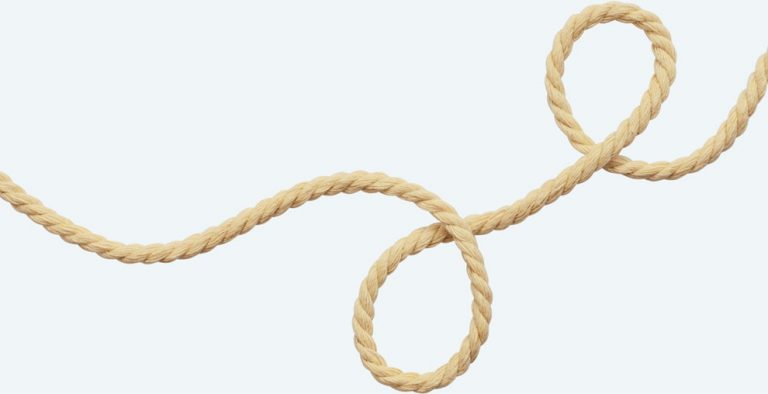Hanging RopeTwo ends of a rope are fixed to the tops of two poles standing straight above a flat ground surface. The poles are unequal in height, with one pole being twice as tall as the other. When the rope is taut and forms a straight line between the tops of the poles, then its length is equal to the sum of the heights of the two poles. What is the approximate maximum possible percentage increase in the rope’s length, relative to its length when taut, so that its lowest hanging point will still be no lower than the top of the shorter pole? Also, what is the approximate minimum percentage increase in the rope’s length, relative to its length when taut, so that its lowest hanging point just barely touches the ground?

Public Key DecryptionHere is a solution is based on Jon Constable’s submission to the March-April 2018 puzzle. In this problem, the encrypted message α = 69,176,418,906,672,230 is of the form x3,038,795,305 mod 2,853,926,939,827,803,391. Furthermore, x = an•27n + an−1•27n−1 +…+ a1•27 + a0, where ai ε {0, 1 ,2,…, 25, 26} corresponding to a blank space for 0 together with the order of the letters of the alphabet.

Let e = 3,038,795,305 and N = 2,853,926,939,827,803,391. Observe, through some computer assistance, that N is the product of two prime numbers, namely p = 1,400,305,337 and q = 2,038,074,743. Let φ be the Euler Totient function and recall that φ(N) = φ(pq) = (p−1)•(q−1) = 2,853,926,936,389,423,312.

Observe gcd(e,φ(N)) = 1. To undo the modular arithmetic, we must determine the multiplicative inverse of e modulo φ(N). Let this be denoted by d. Using computer assistance, we ﬁnd d = 432,954,680,896,144,041. We now determine x by computing αd mod N, where x and α are deﬁned in the statement of the problem. We conclude α = 2,133,862,917,804,125,260.

In base 27 α is represented as {14, 5, 23, 0, 25, 15, 18, 11, 0, 3, 9, 20, 25}. The message decodes to “NEW YORK CITY.”

Solutions were also submitted by Kristen Fox-Neff, Clive Keatinge, Kenneth Klinger, Eamonn Long, Zachary R. Luety, Sean Moore, Chris Norman and John Robertson.